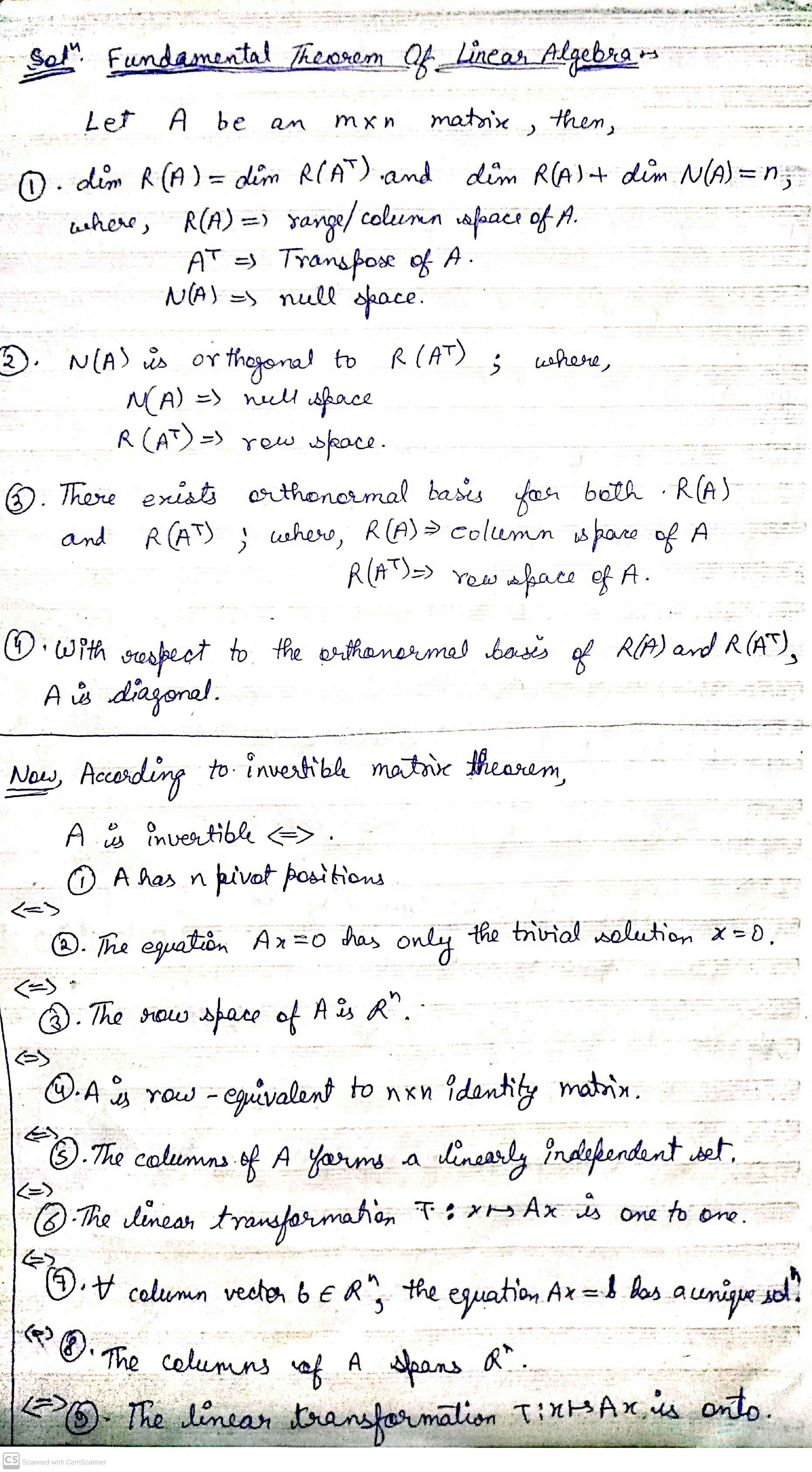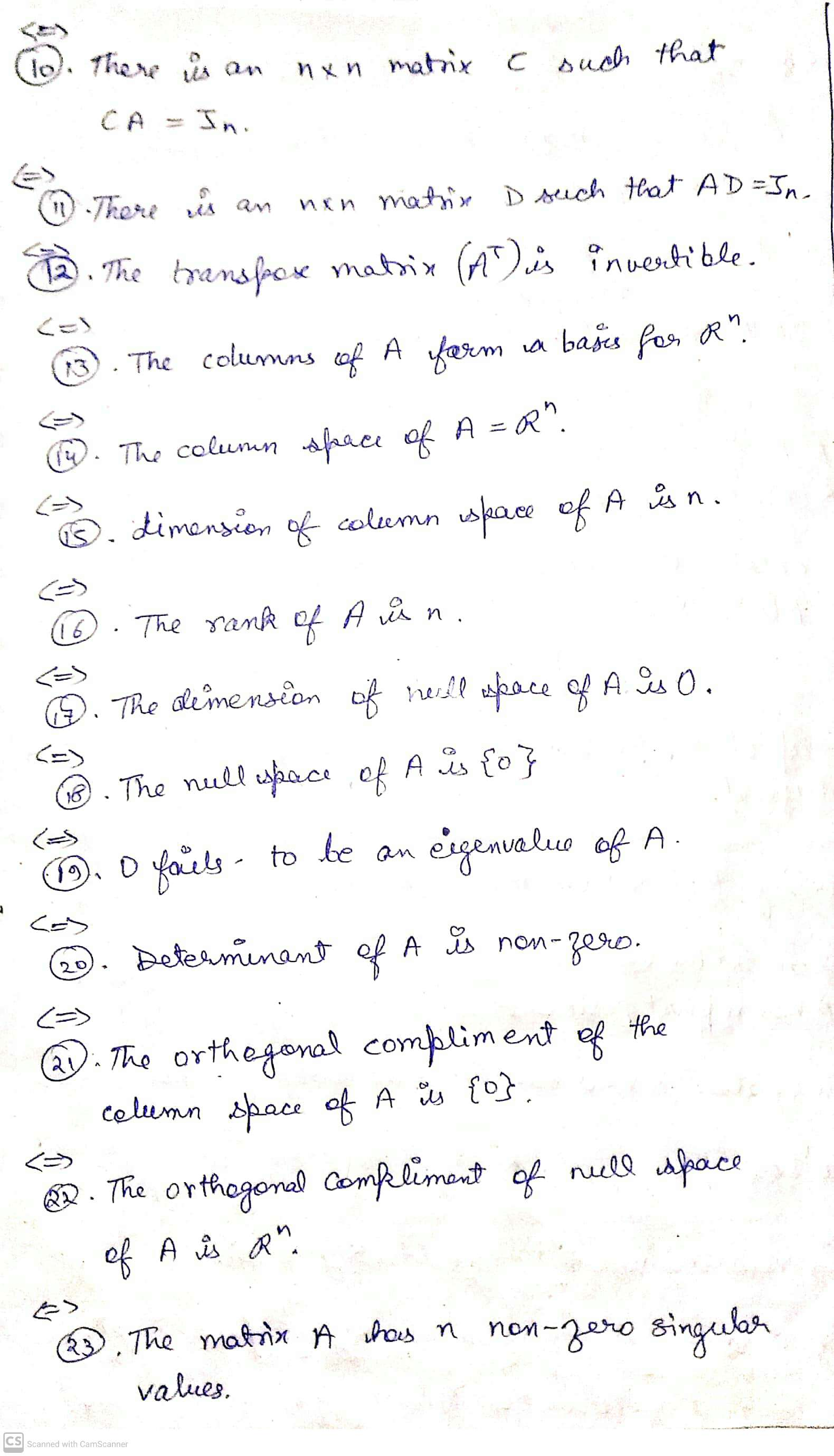# list as many of the parts of fundamental theorem of linear algebra as you can. An...

list as many of the parts of fundamental theorem of linear algebra as you can. An nxn matrix A is invertible if and only if ....

## Solutions

##### Expert SolutionThe answer is in the pic. If any doubt still remained, let me know in the comment section. If this solution helped, please upvote to encourage us. Thanks :)

## Related Solutions

##### What are the two parts of the Fundamental Theorem of Linear Algebra?
What are the two parts of the Fundamental Theorem of Linear Algebra? How can they be applied? Give 1 example problem for each part.
##### fundamtal theorem of linear algebra for dimensions of the fundamental subspace of A
fundamtal theorem of linear algebra for dimensions of the fundamental subspace of A
##### Abstract Algebra and Galois Theory Show how to one can deduce the Fundamental Theorem of Galois...
Abstract Algebra and Galois Theory Show how to one can deduce the Fundamental Theorem of Galois theory from the Artin's lemma.
##### According to the Fundamental Theorem of Algebra, every nonconstant polynomial f (x) ∈ C[x] with complex...
According to the Fundamental Theorem of Algebra, every nonconstant polynomial f (x) ∈ C[x] with complex coefficients has a complex root. (a) Prove every nonconstant polynomial with complex coefficients is a product of linear polynomials. (b) Use the result of the previous exercise to prove every nonconstant polynomial with real coefficients is a product of linear and quadratic polynomials with real coefficients.
##### Prove by induction that it follows from Fundamental Theorem of Algebra that every f(x) ∈ C[x]
Prove by induction that it follows from Fundamental Theorem of Algebra that every f(x) ∈ C[x] can be written into a product of linear polynomials in C[x].
##### Linear algebra
(a) Are there matrices A,B∈Mn(R)A,B∈Mn(R) such that AB−BA=IAB−BA=I. (b) Suppose that A,B∈Mn(R)A,B∈Mn(R) such that (AB−BA)2=AB−BA(AB−BA)2=AB−BA. Show that AA and BB are commutable.
##### Linear algebra Matrix
Let A ∈ Mn(R) such that I + A is invertible. Suppose that                                     B = (I − A)(I + A)-1(a) Show that B = (I + A)−1(I − A)(b) Show that I + B is invertible and express A in terms of B.
##### Linear algebra matrix
Exercise 13. Let A = (aij)n ∈ Mn(R) where aij = cos(i + j) for i, j = 1, 2, . . . ,n. Find rank(A).
##### Linear algebra Matrix
Exercise 11. Find the rank of matrix A where A, B and C
##### Linear algebra Determinant
Exercise 2. In S8, write the following permutations into cyclic form, then determine their signature.(a) 85372164                  (b) 87651234                     (c) 12435687